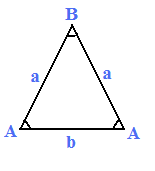## 等腰（等边）三角形在线计算器边a 边b 角A 角B
 面积： 周长： 外接圆半径： 内切圆半径： a边的高： b边的高： a边角平分线： b边角平分线： a边中分线： b边中分线：

 面积(K) K2 = b2 * (4 × a2 - b2) / 16 周长 2 × a + b 边b角平分线 ( tb ) tb2 = 4 × a2 - b2 / 2 边a角平分线( tb ) ta2 = b2 × a × (2a + b) / (a + b)2 边a中位线 ( ma ) ma2 = (2 * b2 + a2) / 4 边b中位线 ( mb ) mb2 = (4 * a2 - b2) / 4 外接圆半径 a / ( 2 * sin (A)) or b / ( 2 * sin (B)) 内切圆半径 (r) r2 = b2 × (2a - b) / (4 × (2a + b))